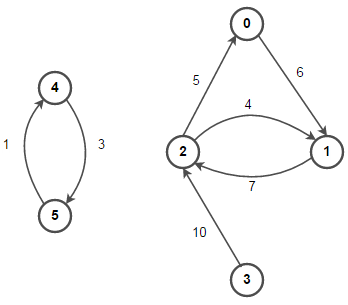# Graph Implementation in C++ using STL

Given an undirected or a directed graph, implement graph data structure in C++ using STL. Implement for both weighted and unweighted graphs using Adjacency List representation of the graph.

Prerequisite: Terminology and Representations of Graphs

As we already know that adjacency list associates each vertex in the graph with the collection of its neighboring vertices or edges i.e every vertex stores a list of adjacent vertices. There are many variations of adjacency list representation depending upon the implementation.For example, below is adjacency list representation of above graph –Above representation allows the storage of additional data on the vertices but practically very efficient when the graph contains only few edges. We will use STL vector class to implement Adjacency List representation of a graph.

Output:

0 –> 1
1 –> 2
2 –> 0 1
3 –> 2
4 –> 5
5 –> 4

#### 2. Weighted Directed Graph implementation using STL –

We know that in a weighted graph, every edge will have a weight or cost associated with it as shown below:Below is C++ implementation of a weighted directed graph using STL. The implementation is similar to above implementation of unweighted directed graph, except here we’ll also store the weight of every edge in the adjacency list.

Output:

(0, 1, 6)
(1, 2, 7)
(2, 0, 5) (2, 1, 4)
(3, 2, 10)
(4, 5, 1)
(5, 4, 3)

Note – We will follow above STL representation of graph as standard for all graph-related problems.

See more:(4 votes, average: 5.00 out of 5)Loading...

Please use our online compiler to post code in comments. To contribute, get in touch with us.
Like us? Please spread the word and help us grow. Happy coding 🙂Subscribe
Notify ofGuest

nice workGuest

simple and conciseGuest
Abhishek

int N = 6
What if there are 7 vertices? What if there are 1002 vertices? Is it a good practice to fix the value of N beforehand?

One of the reasons I prefer techiedelight is because of it’s high-quality solutions which is lacking in other websites. For instance, the code will run despite the`const&` in line number 44, but it’s good coding practice. However, I don’t think fixing the values beforehand is good at all. Please correct me if I am wrongGuest

if you uncomment the line to support undirected graph, then you will find the output is incorrect. Some vertices will be duplicate output.Guest
Suresh Prakash

Well done! 🙂
Here is a generic implementation of the Graphs in C++ using STL https://github.com/sureshprakash/Graph-LibraryGuest

Why is there no nodes in this example?